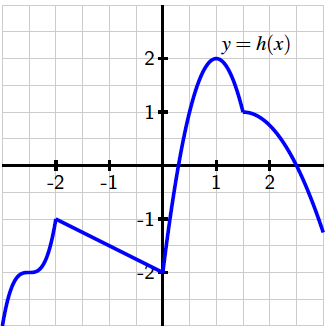The domain of the function $h$ graphed below is all real numbers, and all of its extreme values occur when $-3. Use the graph to answer the following questions.True or false: every relative maximum and minimum of $h$ occurs at a point where $h'(c)$ is either zero or does not exist.

True or false: at every point where $h'(c)$ is zero or does not exist, $h$ has a relative maximum or minimum.

You can earn partial credit on this problem.